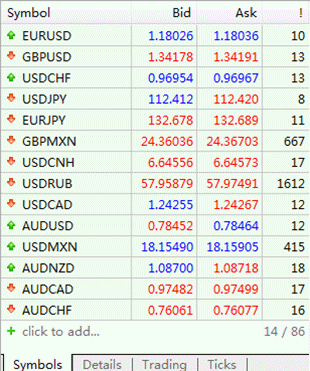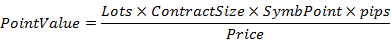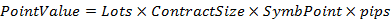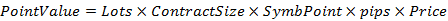Content directory

• Overview
• The concept of point
• Point value
• Output the spread costs of all Forex symbols
• Test
• Conclusion

Overview

Because of the spread, we will have an initial loss when trading any forex currency.The trade cost caused by spreads is an important indicator when formulating trade profit-loss ratios.Spread costs Info are particularly important for short-term traders, especially Scalping traders.This tool can output the spread cost of all Forex currency(including XAU, XAG)  in the platform in different account types (USD account, EUR account, etc.).

In the first part of this paper, the concept of point is introduced. In the second part, the point value and its calculation formula are introduced under the types of dollar account and non-dollar account. The third part introduces the concept of spread cost, and the calculation formula and corresponding code of spread cost. The fourth part describes how to output the spread cost of all Forex symbols in the platform. The fifth part compares the exported spread cost with the spread cost of actual trade and verifies the accuracy of the output data.

The concept of point

The point is the minimum unit of price fluctuations of foreign exchange currency pairs. In systems where the foreign exchange price quote is four digits and two digits, the one-point value represents 0.0001 or 0.01. Currently, in the mainstream foreign exchange dealers platform, the foreign exchange varieties are all 5 and 3 bids. For example, 1 point of EURUSD is 0.00001, and 1 point of USDJPY is 0.001. The chart below shows the buying and selling quotation of some foreign exchange currency pairs.Figure 1. Buy and sell prices and spreads for some Forex symbols

Point Value

Taking into account the five-digit and three-digit quotes are more common, all cases in this article are based on 5 and 3 quotes to explain, other systems of different quotes can be used in the same way, and the MQL5 code written is also applicable to other quotes. system.

A five-quote currency pair, with one-point fluctuation is 0.00001, or a three-quote currency pair, with one-point fluctuation is 0.001, what is the point value they generate per standard lot?

First of all, before calculate the specific point value, we need to determine what the account currency is?

1.  Account currency is USD

Then, consider three situations: 1) a direct currency pair whose base currency is USD; 2) a direct currency pair whose base currency is not USD; and 3) a cross currency pair.

Here are some concepts for a brief explanation: Take EURUSD as an example. The base currency of the currency pair is EUR and the profit currency is USD. In the US dollar account, the direct currency means that the base currency or profit currency is the currency pair of the USD. For example, EURUSD, USDJPY, and cross currency pair means that the base currency and profit currency are not the currency pair of USD, such as EURJPY, EURGBP.

1) a direct currency pair whose base currency is USD

The point value in this case is:(Formula 1)

among them:

• ContractSize：One standard contract size, foreign currency variety is 100,000 base currency
• SymbPoint：the minimum price change for the currency pair (one pip)
• Price：the current price of the currency pair

Take USDJPY as an example, a standard lot fluctuates by one point, Lots=1, ContractSize=100,000 (USD), MinPoint=0.001 (JPY/USD), pips=1, Price is the current price of USDJPY.

Referring to FIG. 1, assuming that the USDJPY price is 112.410 (JPY/USD) at this time, the point value of one standard lot USDJPY fluctuating by one point is 0.89 (USD).

2) direct currency pair whose base currency is not USD

For example: EURUSD, GBPUSD, etc.

The point value in this case is:(Formula 2)

Taking EURUSD as an example, a standard lot fluctuates by one point, Lots=1, ContractSize=100,000 (EUR), SymbPoint=0.00001 (USD/EUR), and pips=1.

Then, the point value at which a standard lot EURUSD fluctuates by one point is 1 (USD).

3) a cross currency pair

For example: EURJPY, EURGBP, etc. There are two scenarios for the calculation of a cross currency pair:

• The direct currency pair currency of the profit currency is USD, the example currency is EURJPY, the profit currency is JPY, the direct currency pair is USDJPY, and the basic currency of USDJPY is USD, the point value in this case is:(Formula 3)

Among them: Price is the current price of the direct currency pair of the profit currency.

Taking EURJPY as an example, a standard lot fluctuates by one point, Lots=1, ContractSize=100,000 (EUR), SymbPoint=0.001 (JPY/EUR), pips=1, and Price is the current price of USDJPY.

Referring to Fig. 1, assuming that the USDJPY price is 112.410 (JPY/USD) at this time, the point value at which a standard hand EURJPY fluctuates by one point is 0.89 (USD).

• The direct currency pair of the profit currency is non-USD, such as EURGBP, and the profit currency is GBP. The direct currency pair is GBPUSD, and the base currency of GBPUSD is not USD, the point value in this case is:(Formula 4)

Among them: Price is the current price of the direct currency pair of the profit currency.

Taking EURGBP as an example, a standard lot fluctuates by one point, Lots=1, ContractSize=100,000 (EUR), MinPoint=0.00001 (GBP/EUR), pips=1, Price is the current price of GBPUSD.

Referring to Figure 1, assuming that the GBPUSD price is 1.34178 (USD/GBP) at this time, the point value of a standard hand EURGBP fluctuating by one point is 1.34 (USD).

2.  Account currency is non-US dollar

Here assumes that the account currency is GBP, and the same applies in other cases.

In this case, all GBP currency pairs are direct currency pairs and non-GBP currency pairs are cross currency pairs.

Also consider three situations: 1) a direct currency pair whose base currency is GBP; 2) a direct currency pair whose base currency is non-GBP; 3) the cross currency pair.

1) a direct currency pair whose base currency is GBP

For example: GBPUSD, GBPJPY, GBPCAD, etc.

The point value in this case is:Where: Price is the current price of the currency pair.

Take GBPUSD as an example, one standard lot fluctuates one point, Lots=1, ContractSize=100,000 (GBP), SymbPoint=0.00001 (USD/GBP), pips=1, Price is the current price of GBPUSD.

Referring to Figure 1, assuming that the GBPUSD price is 1.34178 (USD/GBP) at this time, then the point value of one standard lot GBPUSD fluctuates by one point is 0.75 (GBP).

2) a direct currency pair whose base currency is non-GBP

For example: EURGBP and so on.

The point value in this case is:Taking EURGBP as an example, a standard lot fluctuates by one point, Lots=1, ContractSize=100,000 (EUR), SymbPoint=0.00001 (GBP/EUR), and pipps=1.

Then, the point value of a standard hand EURGBP fluctuates by one point is 1 (GBP).

3) the cross currency pair

For example: EURUSD, EURJPY, etc. There are two scenarios for the calculation of a cross currency pair:

• The direct currency of the profit currency is GBP for the base currency, EURUSD for the example, USD for the profit currency, GBPUSD for the direct currency pair and GBP for the GBPUSD, the point value in this case is:Among them: Price is the current price of the direct currency pair of the profit currency.

Taking EURUSD as an example, a standard lot fluctuates by one point, Lots=1, ContractSize=100,000 (EUR), MinPoint=0.00001 (USD/EUR), pips=1, Price is the current price of GBPUSD.

Referring to Fig. 1, it is assumed that the GBPUSD price is 1.34178 (USD/GBP) at this time, then the point value of a standard hand EURUSD is 0.75 (GBP).

• The direct currency of the profit currency is non-GBP for the base currency does not exist. If the currency pair exists, the point value in this case should be:Among them: Price is the current price of the direct currency pair of the profit currency.

Share it with friends: